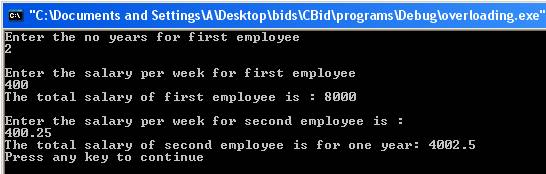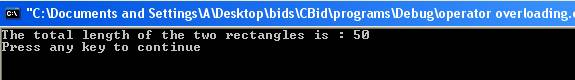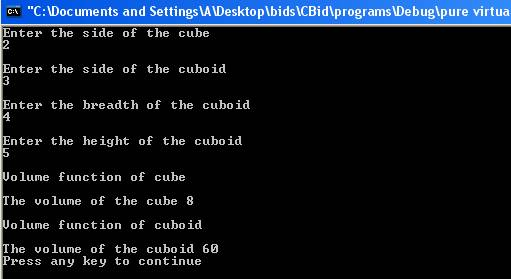Polymorphism and Virtual functions

Polymorphism is defined as one interface to control access to a general class of actions. There are two types of polymorphism one is compile time polymorphism and the other is run time polymorphism. Compile time polymorphism is functions and operators overloading. Runtime time polymorphism is done using inheritance and virtual functions.

#include<iostream>

using namespace std;

class employee

{

public:

int week;

int year;

double calculate(double salary)

{

return(salary*week);

}

int calculate(int salary)

{

return(salary*week*year);

}

employee(int week1)

{

week=week1;

}

employee(int week1,int year1)

{

week=week1;

year=year1;

}

};

int main()

{

int sal;

double sal2;

employee emp1(10);

employee emp2(10,3);

cout << "Enter the no years for first employee" << endl;

cin >> emp1.year;

cout << endl << "Enter the salary per week for first employee" << endl;

cin >> sal;

cout << "The total salary of first employee is : " << emp1.calculate(sal) << endl;

cout << endl << "Enter the salary per week for second employee is : " << endl;

cin >> sal2;

cout << "The total salary of second employee is for one year: " << emp2.calculate(sal2) << endl;

return(0);

}

The result of the program is:-In the program function calculate() and constructor of class employee are overloaded. The function calculate() is declared two times but with different parameter type and same with constructor employee which is also declared two times but with different parameter types. The following statements

double calculate(double salary)

{

return(salary*week);

}

int calculate(int salary)

{

return(salary*week*year);

}

declare functions with same name calculate but one has parameter type integer and other has parameter type double. The function calculate() is overloaded. The statements

employee(int week1)

{

week=week1;

}

employee(int week1,int year1)

{

week=week1;

year=year1;

}

declare two constructors of class employee where one constructor’s parameter list is different from other constructor’s parameter list. The constructor is overloaded. The statement

employee emp1(10);

declares an object emp1 of type employee. When object is created first constructor is called since the argument list matches with parameter list of the constructor. The constructor initializes the data member week of the object emp1. The statement

employee emp2(10,3);

declares an object emp2 of type employee. The second constructor is called since the argument list matches with the parameter list of the constructor. The constructor initializes the data members week and year of the object emp2. In the statement

cout << "The total salary of first employee is : " << emp1.calculate(sal) << endl;

emp1.calculate(sal) calls the first function calculate as the data type of sal matches with the parameter of the function calculate. It displays the total salary which is salary*week*year. In the following statement

cout << "The total salary of second employee is for one year: " << emp2.calculate(sal2) << endl;

emp2.calculate(sal2) calls the second function calculate as the data type of sal2 which is double matches with the data type of parameter of function calculate and hence second function is called. It displays the total salary which is salary*week.

In polymorphism operators can also be overloaded. Operators can be overloaded in order to perform special functions with respect to the class. With the help of operator overloading standard operations such as + , - , * , etc can be applied on the objects of the class. Operators are overloaded by creating operator functions. The keyword operator is used to define operator function. Operator overloading doesn’t allow creating new operators. The general form of operator function is:-

return_type operator #(arg_list)

{

}

return_type is the data type of the value returned from the function. The arg_list is the list of the arguments to the function. The operator comes after the keyword operator. Instead of # there will be an operator. Here is a program to show operator overloading.

#include<iostream>

using namespace std;

class rectangle

{

public:

int length;

{

length=length1;

}

int operator+(rectangle r1)

{

return(r1.length+length);

}

};

int main ()

{

rectangle r1(10,20);

rectangle r2(40,60);

int len;

len=r1+r2;

cout << "The total length of the two rectangles is : " << len << endl;

return(0);

}

The result of the program is:-The program consists of operator +  function  which is overloaded. The + operator is used to perform addition of the length of the objects. The statements

int operator+(rectangle r1)

{

return(r1.length+length);

}

define the operator function whose return type is integer. The operator + is overloaded. The function is used to add the lengths of the two objects. The parameter list consists of one object of type rectangle. The operator()+ takes only one parameter as the operand on the left side of the operator + is passed implicitly to the function through the this operator.  The statement

len=r1+r2;

calls the operator()+ function to calculate the length of the two objects. The return type is of integer. The variable len will contain the total of length of the objects.

Virtual Functions

A virtual function is a member function of the base class and which is redefined by the derived class. When a derived class inherits the class containing the virtual function, it has ability to redefine the virtual functions. A virtual function has a different functionality in the derived class. The virtual function within the base class provides the form of the interface to the function. Virtual function implements the philosophy of one interface and multiple methods. The virtual functions are resolved at the run time.  This is called dynamic binding. The functions which are not virtual are resolved at compile time which is called static binding. A virtual function is created using the keyword virtual which precedes the name of the function.

Virtual functions are accessed using a base class pointer. A pointer to the base class can be created. A base class pointer can contain the address of the derived object as the derived object contains the subset of base class object. Every derived class is also a base class. When a base class pointer contains the address of the derived class object, at runtime it is decided which version of virtual function is called depending on the type of object contained by the pointer. Here is a program which illustrates the working of virtual functions.

include<iostream>

using namespace std;

class shape

{

public:

int side;

virtual int volume()

{

cout << endl <<"Virtual function of base class " << endl;

return(0);

}

};

class cube: public shape

{

public:

int volume()

{

cout << endl << "Volume function of cube " << endl;

return(side*side*side);

}

};

class cuboid:public shape

{

public:

int height;

int volume()

{

cout << endl << "Volume function of cuboid " << endl;

}

};

int main()

{

shape *s,s1;

cube c1;

cuboid c2;

cout << "Enter the side of the cube" << endl;

cin >> c1.side;

cout << endl << "Enter the side of the cuboid " << endl;

cin >> c2.side;

cout << endl << "Enter the breadth of the cuboid" << endl;

cout << endl << "Enter the height of the cuboid" << endl;

cin >> c2.height;

s=&s1;

s->volume();

s=&c1;

cout << endl << "The volume of the cube " << s->volume() << endl;

s=&c2;

cout << endl << "The volume of the cuboid " << s->volume() << endl;

return(0);

}

The result of the program is:-The program has base class shape and class cube and cuboid which inherits base class. The base class has virtual function volume. The statements

virtual int volume()

{

cout << endl <<"Virtual function of base class " << endl;

return(0);

}

define the member function volume() of base class. The function is virtual. The keyword virtual precedes the function return type. In the following statements

int volume()

{

cout << endl << "Volume function of cube " << endl;

return(side*side*side);

}

the derived class cube which inherits base class shape, redefines the function volume() which is virtual. In the following statements

int volume()

{

cout << endl << "Volume function of cuboid " << endl;

}

the derived class cuboid which inherits the base class shape, redefines the function volume(). The statement

shape *s,s1;

declares a pointer to the base class shape. The statement

s=&s1;

pointer s contains the address of the base class object s1. The statement

s->volume();

calls the function volume of the base class shape as the pointer contains the address of the base class object. The statement

s=&c1;

pointer s now contains the address of the derived class object c1. The statement

cout << endl << "The volume of the cube " << s->volume() << endl;

displays the volume of the cube as s->volume() calls the function volume of the derived class cube. The pointer s contains the address of the object of derived class cube. In the statement

s=&c2;

pointer s now contains the address of the derived class object c2. The statement

cout << endl << "The volume of the cuboid " << s->volume() << endl;

displays the volume of the cuboid as s->volume() calls the function volume of the derived class cuboid. The pointer s now contains the address of the object of derived class cuboid.

When a virtual function is not defined by the derived class, the version of the virtual function defined by the base class is called. When a derived class which contains the virtual function is inherited by another class, virtual function can be overloaded for the new derived class. This means that virtual function can be inherited.

A pure virtual function is a function which contains no definition in the base class. The general form of virtual function is:-

virtual return_type function_name(para_list)=0;

The return_type is the type of the value returned. The function_name is the name of the function and para_list is the parameter list.

Each derived class should contain the definition of virtual functions. If the derived class does not define virtual function then compiler will generate an error. A class which contains one or more pure virtual function is called abstract class. Objects of abstract class cannot be created as it does not contain definition of one or more functions. The pointers of abstract class can be created and the references to the abstract class can be made. Here is a same program which shows how pure virtual function is defined.

#include<iostream>

using namespace std;

class shape

{

public:

int side;

virtual int volume()=0;

};

class cube: public shape

{

public:

int volume()

{

cout << endl << "Volume function of cube " << endl;

return(side*side*side);

}

};

class cuboid:public shape

{

public:

int height;

int volume()

{

cout << endl << "Volume function of cuboid " << endl;

}

};

int main()

{

shape *s;

cube c1;

cuboid c2;

cout << "Enter the side of the cube" << endl;

cin >> c1.side;

cout << endl << "Enter the side of the cuboid " << endl;

cin >> c2.side;

cout << endl << "Enter the breadth of the cuboid" << endl;

cout << endl << "Enter the height of the cuboid" << endl;

cin >> c2.height;

s=&c1;

cout << endl << "The volume of the cube " << s->volume() << endl;

s=&c2;

cout << endl << "The volume of the cuboid " << s->volume() << endl;

return(0);

}

The result of the program is:-The statement

virtual int volume()=0;

declares pure virtual function volume() which has no definition. The class shape is now abstract class and objects of type shape cannot be created.

Go to the previous lesson or proceed to the next lesson